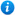Year 3 Fractions Bundle

Year 3 Fractions Bundle

Year 3 maths programme of study - Number - fractions:

- count up and down in tenths; recognise that tenths arise from dividing an object into 10 equal parts and in dividing one-digit numbers or quantities by 10

- recognise, find and write fractions of a discrete set of objects: unit fractions and non-unit fractions with small denominators

- recognise and use fractions as numbers: unit fractions and non-unit fractions with small denominators

- recognise and show, using diagrams, equivalent fractions with small denominators

- add and subtract fractions with the same denominator within one whole

- compare and order unit fractions, and fractions with the same denominators

- solve problems that involve all of the above

This year 3 maths bundle introduces pupils to fractions as per the curriculum objectives of the year 3 maths programme of study listed above. It contains 5 PowerPoint presentations with accompanying worksheets. These include:

Tenths - Year 3

Fractions of Amounts - Year 3

Equivalent Fractions - Year 3

Adding and Subtracting Fractions - Year 3

Comparing and Ordering Fractions - Year 3

For a full description of each resource please click on the image of the included resources below.

Our Price : £7.99 / 10 CreditsResources included (5)

£3.99
Adding and Subtracting Fractions - Year 3
48 powerpoint slides | 7 worksheets
£3.99
Comparing and Ordering Fractions - Year 3
68 powerpoint slides | 11 worksheets
£3.99
Equivalent Fractions - Year 3
59 powerpoint slides | 15 worksheets
£3.99
Fractions of Amounts - Year 3
108 powerpoint slides | 14 worksheets
£2.50
Tenths - Year 3
45 powerpoint slides | 3 worksheets

By continuing to use the site, you agree to the use of cookies. You can change this and find out more by following this link